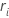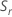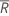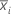# Methods and formulas for estimating sigma for R Chart

The process standard deviation is also called sigma, or σ. If you enter an historical value for sigma, then Minitab uses the historical value. Otherwise, Minitab uses one of the following methods to estimate sigma from the data.

## Rbar method

Minitab uses the range of each subgroup,, to calculate, which is an unbiased estimator of σ:

where

When the subgroup size is constant, the formula simplifies to the following:

where(Rbar) is the mean of the subgroup ranges, calculated as follows:

### Notation

TermDescription
rirange for subgroup i
m number of subgroups
d2(·)value of unbiasing constant d2 that corresponds to the value specified in parentheses.
ninumber of observations in subgroup i
d3(·)value of unbiasing constant d3 that corresponds to the value specified in parentheses.

## Pooled standard deviation method

The pooled standard deviation (Sp) is given by the following formula:

When the subgroup size is constant, Sp can also be calculated as follows:

### With unbiasing constant

By default, Minitab applies the unbiasing constant, c4(), when you use the pooled standard deviation to estimate σ:

When the subgroup size is constant, the unbiased Sp can also be calculated as follows:

### Notation

TermDescription
xijjth observation in the ith subgroupmean of subgroup i
ninumber of observations in subgroup i
μvmean of the subgroup variances
c4(·)value of the unbiasing constant c4 that corresponds to the value that is specified in parentheses.
ddegrees of freedom for Sp, given by the following formula:

## Unbiasing constants d2(), d3(), and d4()

d2(N) is the expected value of the range of N observations from a normal population with standard deviation = 1. Thus, if r is the range of a sample of N observations from a normal distribution with standard deviation = σ, then E(r) = d2(N)σ.

d3(N) is the standard deviation of the range of N observations from a normal population with σ = 1. Thus, if r is the range of a sample of N observations from a normal distribution with standard deviation = σ, then stdev(r) = d3(N)σ.

Use the following table to find an unbiasing constant for a given value, N. (To determine the value of N, consult the formula for the statistic of interest.)

For values of N from 51 to 100, use the following approximation for d2(N):
For values of N from 26 to 100, use the following approximations for d3(N) and d4(N):
• D. J. Wheeler and D. S. Chambers. (1992). Understanding Statistical Process Control, Second Edition, SPC Press, Inc.
• H. Leon Harter (1960). "Tables of Range and Studentized Range". The Annals of Mathematical Statistics, Vol. 31, No. 4, Institute of Mathematical Statistics, 1122−1147.
Table 1. Table of values
N d2(N) d3(N) d4(N)
2 1.128 0.8525 0.954
3 1.693 0.8884 1.588
4 2.059 0.8798 1.978
5 2.326 0.8641 2.257
6 2.534 0.8480 2.472
7 2.704 0.8332 2.645
8 2.847 0.8198 2.791
9 2.970 0.8078 2.915
10 3.078 0.7971 3.024
11 3.173 0.7873 3.121
12 3.258 0.7785 3.207
13 3.336 0.7704 3.285
14 3.407 0.7630 3.356
15 3.472 0.7562 3.422
16 3.532 0.7499 3.482
17 3.588 0.7441 3.538
18 3.640 0.7386 3.591
19 3.689 0.7335 3.640
20 3.735 0.7287 3.686
21 3.778 0.7242 3.730
22 3.819 0.7199 3.771
23 3.858 0.7159 3.811
24 3.895 0.7121 3.847
25 3.931 0.7084 3.883
N d2(N)
26 3.964
27 3.997
28 4.027
29 4.057
30 4.086
31 4.113
32 4.139
33 4.165
34 4.189
35 4.213
36 4.236
37 4.259
38 4.280
39 4.301
40 4.322
41 4.341
42 4.361
43 4.379
44 4.398
45 4.415
46 4.433
47 4.450
48 4.466
49 4.482
50 4.498

## Unbiasing constants c4() and c5()

### Notation

TermDescription
Γ()gamma function
By using this site you agree to the use of cookies for analytics and personalized content.  Read our policy